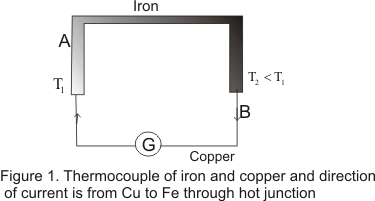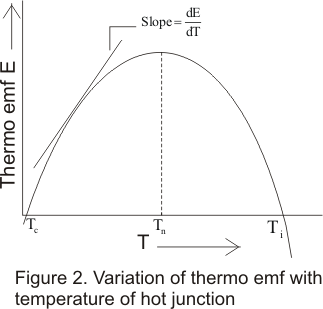# Seebeck effect

## Seebeck effect

• Seebeck effect was first discovered by Thomas John Seebeck
• It stated that when two different conductor are joined to form a circuit and the two junctions are held at the different temperature then an emf is developed which results in the flow of the electric current through the circuit.Arrangement is shown as below in figure
• Magnitude of thermo-electric emf depends upon the nature of the two metals and on the temperature difference between terminals• Seaback effect is reversible i.e, if the hot and cold junctions are reversed the direction of thermoelectric current is also reversed
• Seaback investigated thermo-electric properties of a large number of metals and arranged them in a series known as thermo-electric series or Seeback series and is given as follows

Bi,Ni,Co,Pt,Cu,Mn,Hg,Pb,Sn,Au,Ag,Zn,Cd,Fe,As,Sb,Te
• When any two of these metals in the series is used to form a thermocouple ,the thermo emf is greater when two metals used are farther apart in the circuit
• Figure 1 shows the thermocouple of Cu and Fe.The current in this couple flows from Cu to Fe through the hot junction
• The thermo emf of this couple is only 1.3 mili-volt for a temperature difference of 100 C between the hot and cold junction

## variation of thermo-emf with temperature

• To study the effect of difference of temperature of the two junctions consider a thermo-couple of two dis-similar metals A and B
• Now consider that cold junction is at temperature 0 C and the temperature of the hot junction is raised gradually
• It is found in experiment that thermo emf varies with the temperature of the hot junction
• Figure below shows the graph of variation of thermo emf in the circuit with the variation of temperature of the hot junction.• From graph it is clear that thermo-emf is zero when both the junctions are at the same temperature 0 C and gradually increases as the temperature of the hot junction increases
• The temperature of the hot junction at which thermo-emf in the thermocouple becomes maximum is called neutral temperature (Tn) for that thermocouple
• For a given thermo-couple of two metals neutral temperature has a fixed value
• On further increasing the temperature of the hot junction,after Tn has reached,The thermo-emf decreases and becomes equal at a particular temperature called temperature of inversion Ti.
• Beyond Ti ,if the temperature of hot junction is still increased ,the thermo-emf again started to increase but in reverse direction
• Temperature of inversion Ti is as much above the neutral temperature as neutral temperature is above the temperature of the cold junction.Thus mathematically
Tn-Tc=Ti -Tn
or Tn=(Ti+Tc)/2
Hence neutral temperature is the mean of the temperature of inversion and temperature of the cold junction
• Thermo-emf is the property of each material and can be easily measured for a junction of two dissimilar metals at different temperatures
• Thermo-emf of number of thermocouples is given by the simple relation
E=αT+βT2
where T is the temperature difference between the two junction and α and β are the parameters of the material used
• Also the rate of change of thermo-emf with temperature i.e dE/dT is called thermo-power or Seebeck coefficient S .Mathematically
S=dE/dT